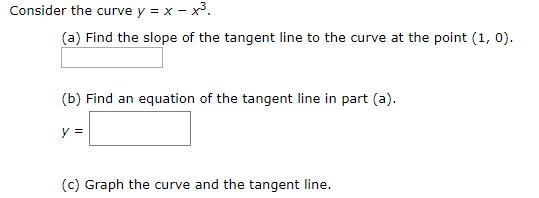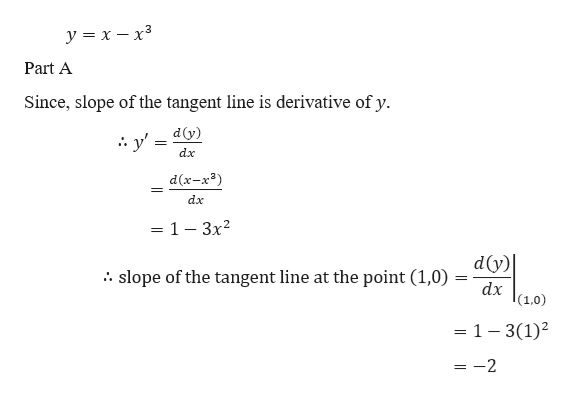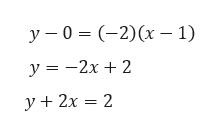# Consider the curve y = x - x*(a) Find the slope of the tangent line to the curve at the point (1, 0)(b) Find an equation of the tangent line in part (a)у 3(c) Graph the curve and the tangent line.

Questionhelp_outlineImage TranscriptioncloseConsider the curve y = x - x* (a) Find the slope of the tangent line to the curve at the point (1, 0) (b) Find an equation of the tangent line in part (a) у 3 (c) Graph the curve and the tangent line. fullscreen
check_circleExpert Solution
Step 1

Given,help_outlineImage Transcriptionclosey x -x3 Part A Since, slope of the tangent line is derivative of y dy) y' dx d(x-x3) dx = 1 - 3x2 dy) dx 10) slope of the tangent line at the point (1,0) . - 1 - 3(1)2 =-2 fullscreen
Step 2

Part B

So, equation of the tangent line having slope -2 and passing through the point (1,0) ishelp_outlineImage Transcriptioncloseу - 0 %3D (-2)(х — 1) у %3 — 2х + 2 У у+ 2х — 2 fullscreen
Step 3

Part C

Graph of the curve and tang...

### Want to see the full answer?

See Solution

#### Want to see this answer and more?

Solutions are written by subject experts who are available 24/7. Questions are typically answered within 1 hour*

See Solution
*Response times may vary by subject and question
Tagged in

### Calculus